# CFD Analysis of Heat Transfer Phenomena in Arc Plasma Reactor

CFD Analysis of Heat Transfer Phenomena in Arc Plasma Reactor

Djamel Eddine GourariBenameur Benameur Giulio Lorenzini Younes Menni

Department of Technology, University Center Salhi Ahmed Naama (Ctr. Univ. Naama), P.O. Box 66, Naama 45000, Algeria

Department of Industrial Engineering, University of Parma, Parco Area delle Scienze 181/A, Parma 43124, Italy

Corresponding Author Email:
gourari@cuniv-naama.dz
Page:
127-132
|
DOI:
https://doi.org/10.18280/i2m.210401
10 January 2022
|
Accepted:
16 March 2022
|
Published:
31 August 2022
| Citation

OPEN ACCESS

Abstract:

In order to control flows and temperature distribution within plasma reactors experimentally requires significant research and development costs. the computational fluid dynamics (CFD) can be used as a powerful tool to investigate the flow pattern and heat transfer inside plasma reactors allowing us to construct best designs with minimal cost. In this work temperature profile and gas flow distribution in the thermal plasma reactor used for carbon nanotubes synthesis is studied by COMSOL Multiphysics. Two background gases Helium (He) and Nitrogen (N2) was compared. We conclude that unlike Nitrogen, Helium ensures a good recirculation in the arc chamber and therefore a good replenishment of the atmosphere by the reactants during the process.

Keywords:

CFD, plasma, arc discharge, simulation, Fluid flow, nanotubes

1. Introduction

Thermal plasmas are widely used for the synthesis of nanomaterials [1-3], arc welding, cutting of metallic materials by plasma torch , plasma spraying , high and medium voltage circuit breakers , arc furnaces in metallurgy, high pressure lamps or vitrification and waste treatment . Consequently, they are the subject of numerous research studies aiming to gain a better understanding of them and to anticipate the physical process that they generate.

The thermal plasma process offers a lot of advantages, such as, enhanced kinetics because of plasma and gaseous state reactions, lower activation energy in synthesizing and high purity because of its clean atmosphere . However, there are many technical obstacles to the full-scale use of plasma process. Firstly, the inhomogeneities of plasma flows make material processing very dependent on the trajectory of the flow, which is difficult to control, and often the flow velocities are high and greatly limit the time of stay in the hot zones. In addition, plasma reactors usually use noble gases, Helium or argon, which increase their cost .

The control of flows and temperature distribution within plasma reactors is a great challenge and experimentally requires significant research and development costs. However, the computational fluid dynamics (CFD) can be used as a powerful tool to investigate the flow pattern and heat transfer inside plasma reactors to gain a better understanding of the physical phenomena at minimal cost.

In this work, a CFD study of a DC arc thermal plasma reactor is performed using the COMSOL tool. The reactor (shown in Figure 1), described in detail elsewhere [2, 3, 10] was developed for the Synthesis of carbon nanotubes, Briefly, it consists of a cylindrical vessel of about 30 cm in diameter and about 0.5 m in height, with two opposed quartz windows placed in front of the plasma and allowing observation of the arc and carrying out a spectroscopic study. The electrodes (two pure graphite rods of 6 mm in diameter) are placed in a vertical configuration to ensure the symmetry of the arc column. The reactor has two valves, one for the primary vacuum (10-1Pa) inside the arc chamber, and the other for the gas filling at the desired working pressure. The volume chosen for the simulation is 18 L at a pressure of 60 kPa.

## 1.png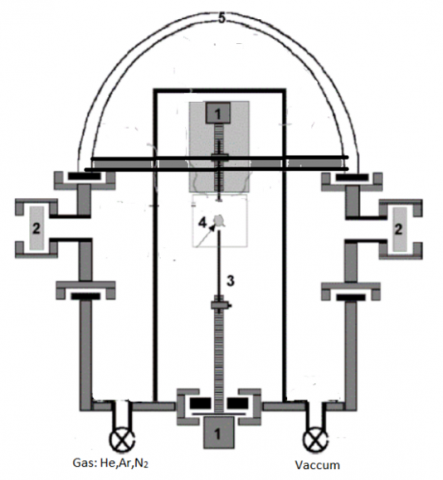1 Step-by-step motors, 2 Quartz window for optical spectroscopy,  3 electrodes, 4 Plasma zone, 5 Bell jar

Figure 1. Sketch of the plasma reactor [2, 11]

2. Geometry and Materials

The reactor studied in this work has a cylindrical geometry with axis symmetry, as well as physical symmetry of the boundary conditions. These two symmetries allow us to reduce the three-dimensional (3D) problem, to a 2D axisymmetric one, using same dimension parameters for the real plasma reactor as illustrated in Figure 2. To ensure geometrical similarity for modeling. The thermal plasma reactor is mainly constructed from solid materials: aluminum for the arc chamber; copper for electrode holders and graphite electrodes.

## 2.pngFigure 2. 2D Axisymmetric geometry and dimensions for the simulated plasma reactor

In the temperature range considered, the thermophysical properties of these materials showed a non-temperature-dependent behavior (no direct contact with the plasma except the graphite electrodes); therefore, the properties of these materials are assumed constant as illustrated in Table 1. However, due to the wide range of temperatures encountered in the plasma reactor by the background gas (Argon; Helium and Nitrogen), temperature dependent properties are required in the CFD simulations. In particular mass density, specific heat, thermal conductivity, dynamic viscosity. They were taken from [12-14].

Table 1. Overview of Solid properties used in the CFD model 

 Solid Material Properties Density (ρ) kg/m3 Specific heat (cp) j/Kg.K Thermal conductivity (k) w/m.K glass ρgl=32 Cpgl=835 kgl=0.038 Copper ρc=8933 Cpc=385 kc=401 Aluminum ρAl=2702 CpAl=903 kAl=237 Graphite  ρel(T) Cpel(T) kel(T)
3. Plasma Gas

The choice of the plasma gas (background gas) plasma is crucial for the quality and yield of the obtained materials. In the literature, Helium is widely used as a plasma gas for the synthesis of nanotubes and other carbonaceous materials by arc [16, 17]. Introducing any other gas into the system seems to strongly affect the plasma state, which is itself responsible for all the hydrodynamics in the reactor, and therefore the yield and the quality of the produced materials [16, 18].

As an example, Figure 3 shows high resolution transmission electron microscopy (HRTEM) images for the synthesized single wall carbon nanotubes in Helium and in Nitrogen for the same experimental conditions, we can see that in Helium, carbon nanotubes are the most dominant carbon phase (tubular structures) on the other hand Nitrogen presents very few nanotubes and favors the growth of other carbon nanostructures.

## 3.pngFigure 3. HRTEM images for synthesized nanotubes in the plasma reactor (A: He plasma gas, B: Nitrogen) 

This is why in this work, the behavior of these two gases is studied and the aim of the current CFD study is to compare the flow conditions, Field temperatures and velocity distributions in the whole plasma reactor to perfectly, control the synthesis process with a better understanding of the growth mechanism, and the origin of the drop in the synthesis yield of nanotubes during the use of Nitrogen as a plasma gas compared to Helium which give a significantly higher yield. Knowing that the use of Nitrogen in the synthesis process is a necessity for e.g. The Nitrogen doping of carbon nanostructures for the control of electronic properties [17-19].

4. Mathematical Formulation

In this section, the system of equations describing the arc plasma reactor is introduced. Here, we only focus on the fluid flow and temperature distribution in the plasma reactor and not on the magnetohydrodynamic phenomena within the plasma itself, which is left for later modeling. The plasma was treated as a momentum/energy source domain of predefined shape (the plasma radius=3mm). It was imposed as one of the boundaries conditions shown in Figure 4. in the inter-electrode gap whose temperature values (Tp) are measured by optical emission spectroscopy as detailed in .

To modelling the heat transfer and the dynamic fluid flow phenomena within the arc plasma reactor presented above, the following assumptions were considered:

• The problem is unsteady for a sequence of 2 minutes.
• The problem is axisymmetric.
• The fluid is incompressible.
• The viscosity of the plasma gas is considered to be variable as a function of temperature.
• The flow is laminar.
• The radiative exchange within the reactor is neglected.

The modeling formulation can be divided into five major elements: plasma gas, electrodes, chamber Walls and the cover. So based on the above assumptions, the governing equations for physical phenomena within the whole reactor can be expressed in the following form:

1. Plasma gas: (He, Ar and N2)

The plasma gas shows natural convection where the equations are:

1. The continuity equation

$\frac{1}{r} \frac{\partial\left(r v_r\right)}{\partial r}+\frac{\partial\left(v_z\right)}{\partial z}=0$           (1)

1. The Navier-Stocks equations

$\left\{\begin{array}{c}\frac{\partial v_r}{\partial t}+v_r \frac{\partial v_r}{\partial r}+v_z \frac{\partial v_r}{\partial z}= \\ -\frac{1}{\rho_g} \frac{\partial p}{\partial r}+v\left[\frac{\partial}{\partial r}\left(\frac{1}{r} \frac{\partial}{\partial r}\left(r v_r\right)\right)+\frac{\partial^2 v_r}{\partial z^2}\right] \\ \frac{\partial v_z}{\partial t}+v_r \frac{\partial v_z}{\partial r}+v_z \frac{\partial v_z}{\partial z}= \\ -g+\beta g\left(T-T_{\infty}\right)-\frac{1}{\rho_g} \frac{\partial p}{\partial z}+v\left[\frac{\partial}{\partial r}\left(\frac{1}{r} \frac{\partial}{\partial r}\left(r v_z\right)\right)+\frac{\partial^2 v_z}{\partial z^2}\right]\end{array}\right.$                 (2)

1. The Energy equation

$\rho_g C p_g\left(\frac{\partial T}{\partial t}+v_r \frac{\partial T}{\partial r}+v_z \frac{\partial T}{\partial z}\right)$$=\frac{1}{r} \frac{\partial}{\partial r}\left(r k_g \frac{\partial T}{\partial r}\right)+\frac{\partial}{\partial z}\left(k_g \frac{\partial T}{\partial z}\right)$              (3)

1. Electrodes (Graphite)

The electrodes show a phase change, so the energy equation is given as follows:

$\rho_{e l} \frac{\partial h}{\partial t}=\frac{1}{r} \frac{\partial}{\partial r}\left(r k_{e l} \frac{\partial T}{\partial r}\right)+\frac{\partial}{\partial z}\left(k_{e l} \frac{\partial T}{\partial z}\right)$                 (4)

1. Electrodes holders (Copper)

$\rho_c C p_c \frac{\partial T}{\partial t}=\frac{1}{r} \frac{\partial}{\partial r}\left(r k_c \frac{\partial T}{\partial r}\right)+\frac{\partial}{\partial z}\left(k_c \frac{\partial T}{\partial z}\right)$            (5)

1. Chamber walls (Aluminum)

$\rho_{A l} C p_{A l} \frac{\partial T}{\partial t}=\frac{1}{r} \frac{\partial}{\partial r}\left(r k_{A l} \frac{\partial T}{\partial r}\right)+\frac{\partial}{\partial z}\left(k_{A l} \frac{\partial T}{\partial z}\right)$            (6)

1. The cover (glass)

$\rho_{g l} C p_{g l} \frac{\partial T}{\partial t}=\frac{1}{r} \frac{\partial}{\partial r}\left(r k_{g l} \frac{\partial T}{\partial r}\right)+\frac{\partial}{\partial z}\left(k_{g l} \frac{\partial T}{\partial z}\right)$              (7)

## 4.png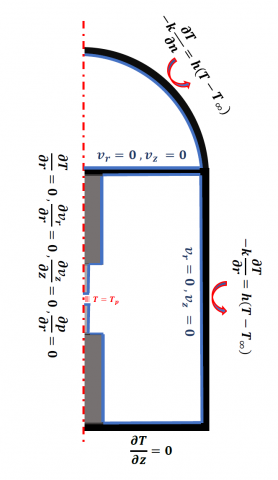Figure 4. Boundry conditions

The numerical simulations for the above equations are performed using a commercially-available software; COMSOL Multiphysics. The COMSOL Multiphysics software utilizes PDEs to model the above physical phenomena. Using the fluid flow and heat transfers modules.

5. Results and Discussion

The influence of the nature of the gas is investigated on the plasma temperature and the velocity distributions. Firstly, these influences are compared in the three cases Helium, Nitrogen and Argon.

5.1 Velocity distribution

The impact of the gas nature on the velocity distribution is discussed from the velocity magnitude profiles at the discharge axis (r = o) and at the plasma edge (r = 3mm).

5.1.1 Z-axis velocity

Figure 5 depicts the axial velocity patterns. The plane z=141 mm correspond to the medium of the inter-electrode gap (hot plasma zone). For the three plasma gases Helium-Nitrogen and Argon, the maximum values (respectively 1 cm.s-1, 14 cm.s-1 and 12 cm.s-1) are reached at the axis of the discharge and present no change in their axial profiles until the plasma edges (r > 3mm) where a velocity pattern change for Helium and Argon is clearly observed on the same figure.

## 5a.png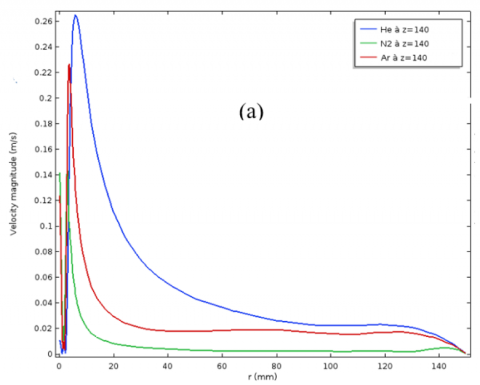## 5b.png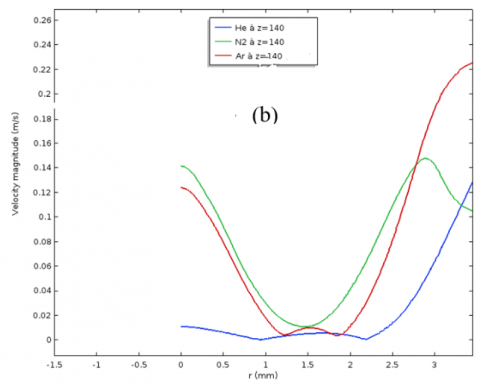Figure 5. Axial profiles velocity for He, N2 and Ar in the middle of the inter-electrode gap (z = 140) mm. (a) in all the reactor, (b) inside plasma (r < 3mm)

This change is reflected in clearly higher velocities compared to those of Nitrogen, which had the maximum velocity inside the plasma. Indeed, the velocity magnitude depends mainly on the density, Helium (Nitrogen) which has the lowest (high) density has the highest (low) velocities. This behavior is reversed within the plasma.

To understand the origin of this reversal, we followed the evolution of the velocity moduli with argon and two other imaginary gases, they have the same properties as argon except that once the dynamic viscosity was replaced by that of Helium and another time the density was changed by that of Helium, thus two argon gases once an argon with a viscosity of Helium and an argon with the density of Helium. This evolution is reported in Figure 6.

We note that the effect of the dynamic viscosity has little influence if we compare the real argon (red curve in Figure 2) and the fictive argon with a viscosity of Helium (cyan curve in Figure 2) where the speed decreases and passes from 0.12 m/s to 0.1 m/s, on the other hand if one compares the real argon with the fictive argon which has the same density as Helium, we notice that the appearance joins that of Helium (blue and purple). the cause of this behavior can be explained by the source of the momentum (mass ratio between Ar and He is equal to 10).

## 6.png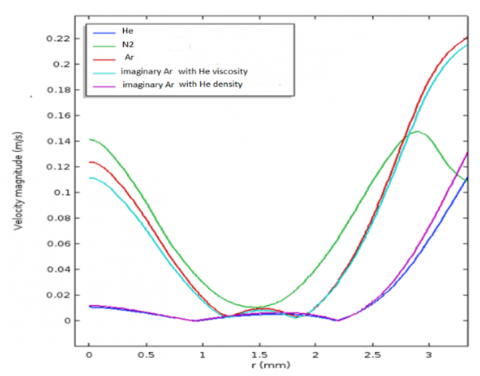Figure 6. Axial profiles velocity for He, N2, Ar and the two imaginary Ar gases gap (z = 140 mm) inside plasma (r <3 mm)

5.1.2 Velocity distributions

The effect of density and the viscosity on the dynamics of the plasma reactor is clearly shown on the velocity distributions for the two gases compared in section 3 i.e. Helium which gives a good yield and Nitrogen which gives a very bad yield of synthesis in nanotubes.

The velocity distributions are shown in Figures 7 and 8. It is noticed that the general aspect of the velocity is very different according to the plasma gas. For Helium the flow field is projected along the axis of the discharge (a predominant z component), this can promote a good recirculation and causes a rise of the gas or reactants escaped at the bottom of the reactor.

The flow field patterns for Nitrogen is shown in Figure 8. (b), one can see that the flow is projected towards the right wall, i.e. a predominant radial component and the presence of "two vortices" at the bottom of the reactor, which may hinder recirculation and therefore the accumulation of gas or reactant at the bottom of the reactor. This zone is therefore poorly replenished by the kinetics and dynamics created by the plasma in the arc chamber.

This may be explained by the difference in the momentum of each gas at the plasma edge.

## 7.png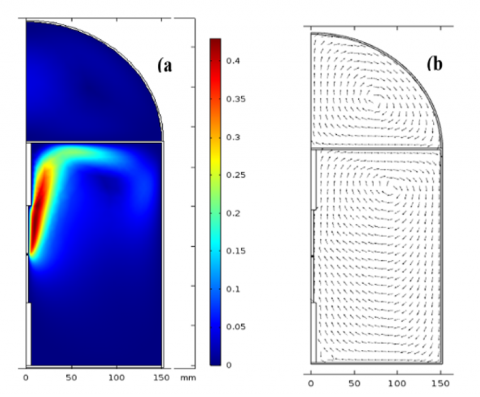Figure 7. (a) Velocity magnitude distribution in He, (b) flow field

## 8.png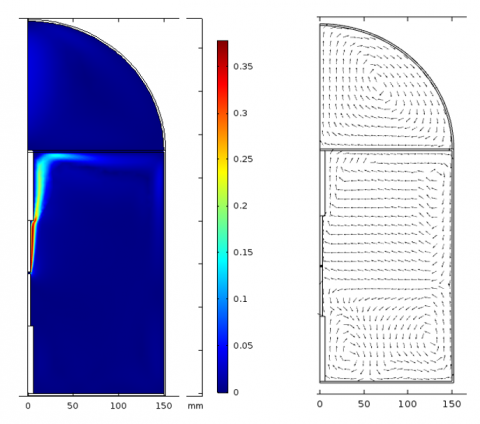Figure 8. (a) Velocity magnitude distribution in N2, (b) flow field

## 9.pngFigure 9. Streamlines in (a) Helium and (b) Nitrogen

5.1.3 Streamlines

Figure 9 shows the streamlines in the arc chamber, we notice that the flow presents a lot of symmetry and thus a good recirculation in Helium. It follows our comments on the velocity fields. Nevertheless, we can notice the presence of a small detachment zone (a small zone on the bottom left of the reactor).

Nitrogen shows two "vortices" at the bottom of the reactor and horizontal lines in the middle and at the top of the chamber, these streamlines are very constricted compared to Helium.

5.2 Temperature distribution

In this section, the temperature fields obtained for each type of plasma gas used are discussed. We remind here that the temperatures within the plasma are imposed.

Figure 10 illustrates the temperature fields, we observe that the temperature distribution follows the plasma shape imposed (at r < 3 mm) this is the same for all the plasma gases used. In the case of Helium we note an axial and especially radial symmetry. This configuration can reflect a better energy distribution in Helium and can be the origin of the ordered convective phenomena present in this gas. The radial symmetry is absent in the case of Nitrogen.

## 10a.png## 10b.png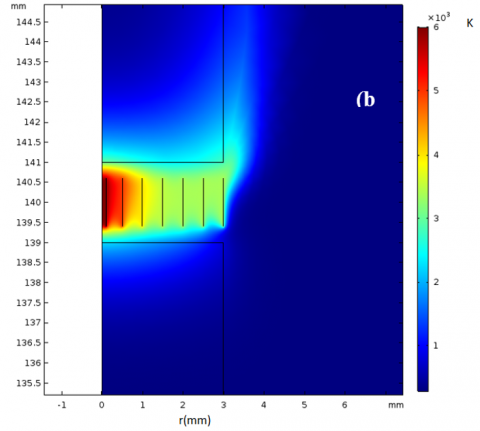Figure 10. Temperature distributions a) He, b) N2

We also note a difference in the morphology of the thermal zone where we observe a "thermal confinement" in Nitrogen reflected by a minimization of the contact zone between plasma and electrodes, this effect is reversed in Helium where there is a "thermal expansion" shown in Figure 8 (a) with a cyan-green color in the area between z = 139 and z = 141 for r > 3mm. These differences in these areas reflect a mechanism of energy transfer from the hot gas to the electrode and this mechanism is specific to each gas.

To explain the difference in the energy transfer mechanism in the gases, we thought of a difference in the thermal conductivity of the gases, so that a gas with low thermal conductivity would have a high thermal resistance and therefore a higher energy deposited on the electrode, This was not the case, in fact in the range of temperatures imposed in the inter-electrode gap, Nitrogen represents the highest thermal conductivity and the thermal conductivity can not justify the thermal behavior of gases.

The only explanation we could make is that our study is conducted in the unsteady regime and the thermal diffusivity of each essential element in the study (gas-solid) was calculated and we could conclude that Nitrogen has the highest thermal volume storage capacity at temperatures > 6000 K ≈52 J/m3.K-1 against ≈37 J/m3.K-1 for Helium therefore on the selected sequence, the temperature rise in the case of the use of Nitrogen cannot be observed.

6. Conclusions

For to the full-scale use of thermal plasmas, in particular the use of synthesis processes, such as electric arc reactors, it is necessary to control all the physical aspects involved in the reactor, so a better understanding of the energy and mass transfer phenomena is necessary. In this work a hydrodynamic and thermal simulation of the reactor has been performed.

An unsteady model is studied, we have demonstrated throughout the work the influence of the parameters that we considered essential (nature of plasma gas, plasma temperature profile) on the flow fields, and the velocity vectors and also we have studied the influence of these parameters on the temperature fields and the streamlines.

We have seen that Helium ensure a good recirculation in the arc chamber and therefore a good replenishment of the atmosphere by the reactants during the process, which was not with Nitrogen where the presence of detachment zones can be present.

Nomenclature
 T Temperature, K Cp Specific heat, J. kg-1. K-1 k Thermal conductivity, W.m-1. K-1 g Gravitational acceleration, m. s-2 p Pressure, N. m-2 v Velocity magnitude, m. s-1 r r coordinate, m z z coordinate, m Tp Imposed plasma temperature h heat convection coefficient, W.m-2.K-1 Greek symbols v Kinematic viscosity, m2. s-1 $\beta$ thermal expansion coefficient, K-1 ρ Density, Kg. m-3 Subscripts r r direction z z direction g Gas el Electrode c Copper Al Aluminium gl Glass
References

 Iijima, S. (1991). Helical microtubules of graphitic carbon. Nature, 354: 56-58. https://doi.org/10.1038/354056a0

 Gourari, D.E., Razafinimanana, M., Monthioux, M., Arenal, R., Valensi, F., Joulie, S., Serin, V. (2016). Synthesis of (B-C-N) nanomaterials by arc discharge using heterogeneous anodes. Plasma Sci. Technol., 18(5): 465-468. https://doi.org/10.1088/1009-0630/18/5/03

 Gourari, D.E. (2015). Synthèse par arc électrique de nanotubes de carbone hybrides incorporant de l’azote et/ou du bore. Université Paul Sabatier (Toulouse 3). https://hal.archives-ouvertes.fr/tel-01813681.

 Trelles, J.P., Chazelas, C., Vardelle, A., Heberlein, J.V.R. (2009). Arc plasma torch modeling. J. Therm. Spray Technol., 18(5): 728-752. https://doi.org/10.1007/s11666-009-9342-1

 Datta, S., Pratihar, D.K., Bandyopadhyay, P.P. (2013). Modeling of plasma spray coating process using statistical regression analysis. Int. J. Adv. Manuf. Technol., 65: 967-980. https://doi.org/10.1007/s00170-012-4232-y

 Randrianarivao, D. (2012). Modélisation des écoulements dans un disjoncteur haute tension. http://www.theses.fr/2012TOU30166/document.

 Sharma, D., Mistry, A., Mistry, H., Chaudhuri, P., Murugan, P.V., Patnaik, S., Sanghariyat, A., Jain, V., Chaturvedi, S., Nema, S.K. (2020). Thermal performance analysis and experimental validation of primary chamber of plasma pyrolysis system during preheating stage using CFD analysis in ANSYS CFX. Therm. Sci. Eng. Prog., 18: 100525. https://doi.org/10.1016/j.tsep.2020.100525

 Li, Y.D., Reddy, R. (2017). Numerical study of the fluid flow and temperature distribution in a non-transferred DC ARC thermal plasma reactor. Miner. Met. Mater. Ser., 445-454. https://doi.org/10.1007/978-3-319-51091-0_43

 Ravary, B. (1998). Modélisation thermique et hydrodynamique d’un réacteur plasma triphasé. Contribution à la mise au point d’un procédé industriel pour la fabrication de noir de carbone. École Nationale Supérieure des Mines de Paris. https://pastel.archives-ouvertes.fr/tel-01138155.

 Mansour, A., Razafinimanana, M., Monthioux, M., Pacheco, M., Gleizes, A. (2007). A significant improvement of both yield and purity during SWCNT synthesis via the electric arc process. Carbon, 45(8): 1651-1661. https://doi.org/10.1016/j.carbon.2007.03.044

 Ramarozatovo, V. (2012). Influence of chamber volume in single-walled carbon nanotube synthesis by an electric arc. Journal of Physics D Applied Physics, 45(34): 345204. https://doi.org/10.1088/0022-3727/45/34/345204

 Boulos, M.I., Fauchais, P., Pfender, E. (2016). Thermal Arcs. In: Handbook of Thermal Plasmas. Springer, Cham. https://doi.org/10.1007/978-3-319-12183-3_12-1

 Murphy, A.B. (1997). Transport coefficients of helium and argon-helium plasmas. IEEE Trans. Plasma Sci., 25(5): 809-814. https://doi.org/10.1109/27.649574

 Wang, W.Z., Rong, M.Z., Murphy, A.B., Wu, Y., Spencer, J.W., Yan, J.D., Fang, M.T.C. (2011). Thermophysical properties of carbon-argon and carbon-helium plasmas. J. Phys. D. Appl. Phys., 44(35). https://doi.org/10.1088/0022-3727/44/35/355207

 Bergman, T.L., Lavine, A.S., Incropera, F.P. (2011). Fundamentals of Heat and Mass Transfer, 7th Edition. John Wiley & Sons. https://books.google.dz/books?id=5cgbAAAAQBAJ.

 Ben Belgacem, A., Hinkov, I., Ben Yahia, S., Brinza, O., Farhat, S. (2016). Arc discharge boron nitrogen doping of carbon nanotubes. Mater. Today Commun., 8: 183-195. https://doi.org/10.1016/j.mtcomm.2016.08.001

 Panchakarla, L.S., Subrahmanyam, K.S., Saha, S.K., Govindaraj, A., Krishnamurthy, H.R., Waghmare, U.V., Rao, C.N.R. (2009). Synthesis, structure, and properties of boron- and nitrogen-doped graphene. Adv. Mater., 21(46): 4726-4730. https://doi.org/10.1002/adma.200901285

 Vonjy, R. (2011). In-situ elaboration and characterization of heterogeneous nanotubes by electric arc plasma. University Paul Sabatier, Toulouse III (Elaboration in-situ et caractérisation de nanotubes hétérogènes par plasma d’arc électrique). http://thesesups.ups-tlse.fr/1948/.

 Monthioux, M. (2012). Carbon Meta-Nanotubes: Synthesis, Properties, and Applications. Wiley-Blackwell. https://hal.archives-ouvertes.fr/hal-02106281.